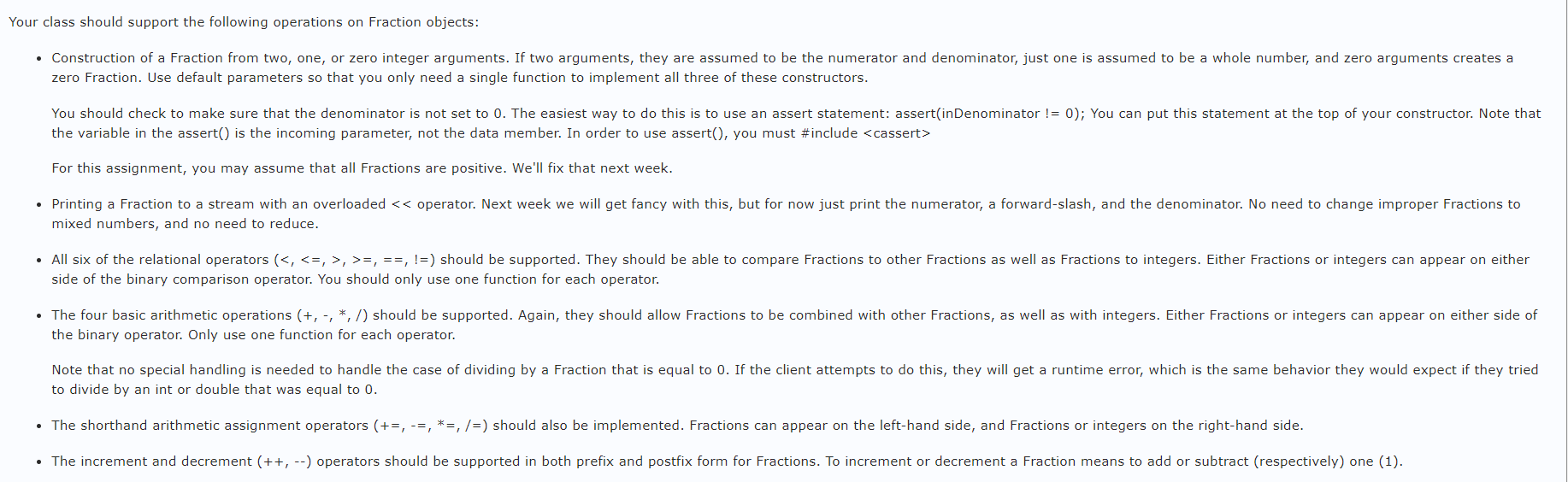# C++ Question Your class should support the following operations on Fraction objects: • Construction of a...

###### Question:

C++ QuestionYour class should support the following operations on Fraction objects: • Construction of a Fraction from two, one, or zero integer arguments. If two arguments, they are assumed to be the numerator and denominator, just one is assumed to be a whole number, and zero arguments creates a zero Fraction. Use default parameters so that you only need a single function to implement all three of these constructors. You should check to make sure that the denominator is not set to 0. The easiest way to do this is to use an assert statement: assertinDenominator != 0); You can put this statement at the top of your constructor. Note that the variable in the assert() is the incoming parameter, not the data member. In order to use assert(, you must #include <cassert> For this assignment, you may assume that all Fractions are positive. We'll fix that next week. • Printing a Fraction to a stream with an overloaded << operator. Next week we will get fancy with this, but for now just print the numerator, a forward-slash, and the denominator. No need to change improper Fractions to mixed numbers, and no need to reduce. • All six of the relational operators (<, <=, >, >=, ==, !=) should be supported. They should be able to compare Fractions to other Fractions as well as Fractions to integers. Either Fractions or integers can appear on either side of the binary comparison operator. You should only use one function for each operator. • The four basic arithmetic operations (+,-- */) should be supported. Again, they should allow Fractions to be combined with other Fractions, as well as with integers. Either Fractions or integers can appear on either side of the binary operator. Only use one function for each operator. Note that no special handling is needed to handle the case of dividing by a Fraction that is equal to 0. If the client attempts to do this, they will get a runtime error, which is the same behavior they would expect if they tried to divide by an int or double that was equal to 0. • The shorthand arithmetic assignment operators (+=, -=, *=, /=) should also be implemented. Fractions can appear on the left-hand side, and Fractions or integers on the right-hand side. • The increment and decrement (++, --) operators should be supported in both prefix and postfix form for Fractions. To increment or decrement a Fraction means to add or subtract (respectively) one (1).